# P4-structure and Its Relatives

The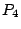-structure of a graph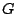is the 4-uniform hypergraph whose vertex-set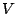is the vertex-set ofand whose hyperedges are the subsets ofthat induce's (chordless paths on four vertices) in. The graph property of being Berge can be formulated directly in terms of-structure: a graph is Berge if and only if its-structure contains no induced odd ring, meaning a 4-uniform hypergraph with vertices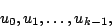where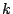is odd and at least five, and with thehyperedges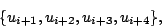where the subscripts are taken modulo. (The if'' part is trivial and the only if'' part is easy, even though a little tedious; see [ MR 86j:05119] for a sketch of the argument.)

Can the Chudnovsky-Robertson-Seymour-Thomas decomposition theorem for Berge graphs be reformulated directly in terms of-structure?

The five classes of basic graphs featured in the theorem lend themselves nicely to such reformulations: there are classes C of 4-uniform hypergraphs such that

• the-structure of every basic graph belongs to C,
• no 4-uniform hypergraph in C contains an induced odd ring,
• membership in C can be tested in polynomial time.
One such class is defined in terms of a certain directed graph,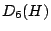, associated with every 4-uniform hypergraph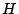: C consists of all 4-uniform hypergraphssuch that
•contains no induced ring with five vertices and
• all strongly connected components ofare bipartite.
The vertices ofare all the ordered 6-tuples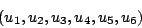of distinct vertices ofsuch that the sub-hypergraph ofinduced by the set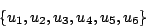consists of the three hyperedges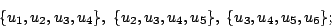there is a directed edge from vertexofto vertex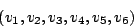ofif and only if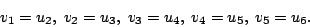Trivially, membership in C can be tested in polynomial time; trivially, no 4-uniform hypergraph in C contains an induced odd ring; a proof that the-structure of every basic graph belongs to C is easy, even though a little tedious ([here] is a sketch of the argument).

The four kinds of structural faults featured in the decomposition theorem suggest the following three problems.

Problem 1: Find a class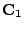of 4-uniform hypergraphs such that

• the-structure of every graph with a 2-join belongs to,
• no odd ring belongs to,
•belongs to NP.

Problem 2: Find a class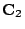of 4-uniform hypergraphs such that

• the-structure of every graph with an M-join belongs to,
• no odd ring belongs to,
•belongs to NP.

Problem 3: Find a class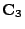of 4-uniform hypergraphs such that

• the-structure of every graph with a balanced skew partition belongs to,
• no odd ring belongs to,
•belongs to NP.

Chính Hoàng introduced additional graph functions that are invariant under complementation and determine whether or not a graph is Berge: these are the co-paw-structure ([ MR 2001a:05065]), the co-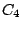-structure (Discrete Math. 252 (2002), 141-159), and the co-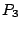-structure (to appear in SIAM J. Discrete Math.). Can the decomposition theorem be reformulated directly in terms of one of Hoàng's invariants?

Contributed by Vašek Chvátal

Back to the main index for Perfect Graphs.